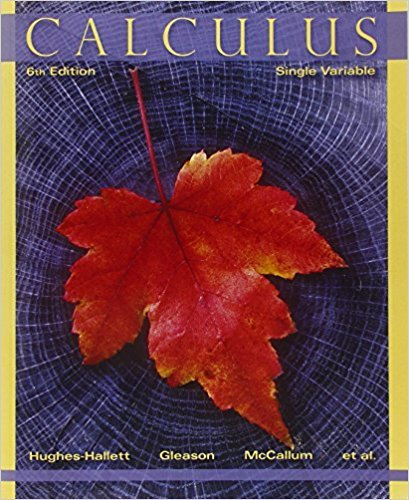×
×

# Solutions for Chapter 4.2: OPTIMIZATION## Full solutions for Calculus: Single Variable | 6th Edition

ISBN: 9780470888643Solutions for Chapter 4.2: OPTIMIZATION

Solutions for Chapter 4.2
4 5 0 337 Reviews
19
1
##### ISBN: 9780470888643

Chapter 4.2: OPTIMIZATION includes 62 full step-by-step solutions. Calculus: Single Variable was written by and is associated to the ISBN: 9780470888643. This expansive textbook survival guide covers the following chapters and their solutions. Since 62 problems in chapter 4.2: OPTIMIZATION have been answered, more than 32420 students have viewed full step-by-step solutions from this chapter. This textbook survival guide was created for the textbook: Calculus: Single Variable , edition: 6.

Key Calculus Terms and definitions covered in this textbook
• Arcsine function

See Inverse sine function.

• Elementary row operations

The following three row operations: Multiply all elements of a row by a nonzero constant; interchange two rows; and add a multiple of one row to another row

• Exponential function

A function of the form ƒ(x) = a ? bx,where ?0, b > 0 b ?1

• Factored form

The left side of u(v + w) = uv + uw.

• Gaussian curve

See Normal curve.

• Initial side of an angle

See Angle.

• kth term of a sequence

The kth expression in the sequence

• Law of cosines

a2 = b2 + c2 - 2bc cos A, b2 = a2 + c2 - 2ac cos B, c2 = a2 + b2 - 2ab cos C

• Multiplicative inverse of a real number

The reciprocal of b, or 1/b, b Z 0

• n factorial

For any positive integer n, n factorial is n! = n.(n - 1) . (n - 2) .... .3.2.1; zero factorial is 0! = 1

• Natural logarithmic function

The inverse of the exponential function y = ex, denoted by y = ln x.

• Root of a number

See Principal nth root.

• Series

A finite or infinite sum of terms.

• Slant asymptote

An end behavior asymptote that is a slant line

• Tangent

The function y = tan x

• Transformation

A function that maps real numbers to real numbers.

• Tree diagram

A visualization of the Multiplication Principle of Probability.

• Union of two sets A and B

The set of all elements that belong to A or B or both.

• Vector equation for a line in space

The line through P0(x 0, y0, z0) in the direction of the nonzero vector V = <a, b, c> has vector equation r = r0 + tv , where r = <x,y,z>.

• Work

The product of a force applied to an object over a given distance W = ƒFƒ ƒAB!ƒ.

×